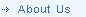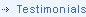Research Research in the topic of large-scale unconstrained nonlinear optimization where the function had a special structure. The research involved developing and analyzing numerically stable algorithms for minimizing a nonlinear function whose Hessian (second derivative) matrix is sparse (has many zero elements). The implementation of these algorithms required a thorough knowledge of software design, data structure and numerical analysis. Some of the situations in which numerical analysis can help include: Determination of the numerical stability of a computer generated solution. That is, is the answer unstable because of the inherent nature of the formulation or because of the scaling of the problem? Determination of the numerical stability of the algorithms. TOX RISK TOX RISK is a software package designed to perform health-related risk assessments. For example, it can be used to estimate the likelihood of developing cancer after exposure to different levels of certain chemicals. The program uses the maximum likelihood method to estimate the parameters associated with the risk. The underlying optimization problem is a nonlinear program with linear constraints. SBSI’s work with TOX RISK involved examination of the numerical properties of the algorithm used by TOX RISK to perform this optimization. The first step in this task was to make sure that the algorithm is stable. This step involved examining the systems of equations that must be solved during the algorithm and verifying that the procedures used to compute their solutions limit the accumulation of round-off error. Otherwise, the computed solution to the optimization problem may be wrong. SBSI staff also checked that the algorithm took precautions against ill-conditioned problems, another potential cause of incorrectly computed solutions. In addition to verifying the stability of TOX RISK’s equation solver, SBSI staff also examined the suitability of the algorithm used to perform the optimization. Information about stability, choice of numerical tolerances and impact of numerical errors are some of the issues that must be dealt with properly to ensure that an algorithm computes a true optimal solution.Commercial Software Disk Drive Related Prospect Evaluation User Interface Tracking Software Mail/Invoice Programs Levedaged Leasing Distribution Systems Miscellaneous Planning Models Scheduling Models Software Design Market Analysis Model Resource Balancing / Site Evaluation Of Systems Desktop Publishing